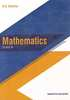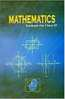CBSE (Arts) Class 11CBSE
Share

# Textbook Solutions for CBSE (Arts) Class 11 - CBSE

< prev 1 to 2 of 2 next >RD Sharma Solutions for Class 11 Mathematics Textbook Mathematics

Chapters : Sets, Relations, Functions, Measurement of Angles, Trigonometric Functions, Graphs of Trigonometric Functions, Values of Trigonometric function at sum or difference of angles, Transformation formulae, Values of Trigonometric function at multiples and submultiples of an angle, Sine and cosine formulae and their applications, Trigonometric equations, Mathematical Induction, Complex Numbers, Quadratic Equations, Linear Inequations, Permutations, Combinations, Binomial Theorem, Arithmetic Progression, Geometric Progression, Some special series, Brief review of cartesian system of rectangular co-ordinates, The straight lines, The circle, Parabola, Ellipse, Hyperbola, Introduction to three dimensional coordinate geometry, Limits, Derivatives, Mathematical reasoning, Statistics, ProbabilityNCERT Solutions for Class 11 Mathematics Textbook Mathematics

Chapters : Sets, Relations and Functions, Trigonometric Functions, Principle of Mathematical Induction, Complex Numbers and Quadratic Equations, Linear Inequalities, Permutations and Combinations, Binomial Theorem, Sequences and Series, Straight Lines, Conic Sections, Introduction to Three Dimensional Geometry, Limits and Derivatives, Mathematical Reasoning, Statistics, Probability

< prev 1 to 2 of 2 next >

## Textbook solutions and Reference book solutions for Class 11

• On this page you will find RD Sharma solution, NCERT solution for Class 11.
• Each chapter comes with subsections that provide in-depth knowledge about the basic concepts.
• Solved equations along with examples help the students to understand the different steps towards solving their exercises.
• Compilation of different exercises makes it a great choice for students to practice to brush up their concepts and skills.
• These books are a must-have for the students who want to closely evaluate the different aspects of their respective subjects.
• Different graphs along with schematic representations help the students to memorise the many steps.
• These solutions for Class 11 are prepared by experts, include the problems from latest edition and are as per CBSE pattern.

## Reference book solutions and textbook solutions on Shaalaa.com

Shaalaa.com is a great source for Reference book solutions and textbook solutions of all Subjects for Practice. Our best teachers have reviewed all the Class 11 solutions of exercise questions that will help you solve all the Class 11 questions of all subject without any problem. Reference book Solutions are highly beneficial especially when you are thinking to prepare for school as well as competitive level examinations. We at Shaalaa.com work hard to create better ways in order to help students and provide you with the detailed solutions for all questions from reference boook and textbook as per the latest marking scheme pattern is to back you up in effective learning in all the examinations conducted by CBSE.
S